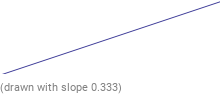Search IntMath
Close

# Angles Between Two Lines in Geometry## What is an Angle?

An angle is a measure of the amount of turn from one line to another. An angle is formed when two lines intersect, or when a line meets itself at a point. In geometry, the measure of an angle is usually expressed in degrees.

## Types of Angles

There are several different types of angles, each with its own particular properties. The most common types of angles are acute angles, right angles, obtuse angles, and straight angles.

An acute angle is an angle that is less than 90 degrees. A right angle is an angle that is exactly 90 degrees. An obtuse angle is an angle that is greater than 90 degrees, but less than 180 degrees. A straight angle is an angle that measures exactly 180 degrees.

## Angles Between Two Lines

When two lines intersect, the angles between them can be classified as either interior angles or exterior angles. Interior angles are angles that are located between the two lines on the inside of the polygon formed by the lines. Exterior angles are angles that are located between the two lines on the outside of the polygon formed by the lines.

When two lines intersect, the sum of the interior angles is always equal to 180 degrees. This is because of the fact that the sum of all the angles in any polygon is always equal to 180 degrees.

## Parallel Lines

When two lines are parallel, they never intersect. This means that there are no angles between the two lines. However, when two lines are parallel, the angles formed by a line that intersects both of the lines are equal. This is because the angles formed by parallel lines are supplementary, which means that they add up to 180 degrees.

## Angles Between Intersecting Lines

When two lines intersect, the angles between them can be classified as either acute, right, obtuse, or straight. This classification is determined by the measure of the angle. If the angle measures less than 90 degrees, it is an acute angle. If the angle measures exactly 90 degrees, it is a right angle. If the angle measures more than 90 degrees but less than 180 degrees, it is an obtuse angle. If the angle measures exactly 180 degrees, it is a straight angle.

When two lines intersect, the angles between them can also be classified as either interior angles or exterior angles. Interior angles are angles that are located between the two lines on the inside of the polygon formed by the lines. Exterior angles are angles that are located between the two lines on the outside of the polygon formed by the lines.

## Practice Problems

1. If two lines intersect at a point, what type of angle will be formed?

2. If two lines are parallel, what type of angle will be formed?

3. What is the sum of all the interior angles in a triangle?

4. What is the sum of the exterior angles in a pentagon?

5. What type of angle is formed if the angle measures 120 degrees?

6. What type of angle is formed if the angle measures 45 degrees?

## Conclusion

In this article, we discussed angles between two lines in geometry. We discussed different types of angles, such as acute angles, right angles, obtuse angles, and straight angles. We also discussed interior and exterior angles, and the fact that the sum of the interior angles of a polygon is always equal to 180 degrees. Finally, we discussed the angles that are formed when two lines are parallel and when two lines intersect.

By understanding angles between two lines in geometry, you will be able to solve a variety of mathematical problems. With practice, you should be able to identify different types of angles and calculate the measures of angles in a variety of situations.

## FAQ

### What is the angle between two lines?

The angle between two lines is the angle formed by the intersection of the two lines at their point of intersection.

### How do you find the angle of a line?

The angle of a line can be found by measuring the angle between the line and the x-axis.

### When two lines intersect and the angle between them is A?

When two lines intersect, the angle between them is the angle formed by the intersection of the two lines at their point of intersection.

## Problem SolverThis tool combines the power of mathematical computation engine that excels at solving mathematical formulas with the power of GPT large language models to parse and generate natural language. This creates math problem solver thats more accurate than ChatGPT, more flexible than a calculator, and faster answers than a human tutor. Learn More.